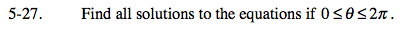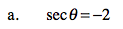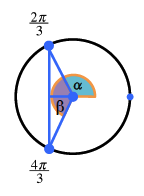### Home > PC > Chapter 5 > Lesson 5.1.2 > Problem5-27

5-27.$\frac{1}{\text{cos}\theta}=-2$

$1=-2\text{cos}\theta$

$-\frac{1}{2}=\text{cos}\theta$Use a similar thinking process as in part (a).